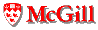# Data Structure Metrics

Last updated: December 4, 2002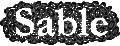Dynamic metrics for program size and structure try to answer the question: how large is a program and how complex is its control structure?

## Array Intensive Metrics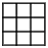Many "scientific" benchmarks are deemed so because the dominant data structures are arrays. The looping and access patterns used for array operations are then expected to provide opportunities for optimization. Determining if a program is array intensive will then be a problem of determining if there are a significant, or dominant number of array accesses. Note that since Java multi-dimensional arrays are stored as arrays of arrays, the number of array access operations required for each multi-dimensional array element is magnified. To avoid skewing results, the type of element located at the array element should be inspected, and if it is an array type it should be discounted.
• data.arrayDensity.value: This is a metric describing the relative importance of array access operations. For uniformity, we express it as the average number of array access operations excluding accesses where the target value is not of array type, per kbc of executed code. A data.arrayDensity.value of greater than 100 indicates an array intensive program. A corresponding data.arrayDensity.continuous metric measures the same quantity incrementally, at various intervals in program execution.

## Floating-Point Intensive Metrics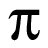Programs that do numerous floating point calculations also tend to be considered "scientific". Different optimizations apply though; including the choice of appropriate math libraries optimized for speed or compatibility, opportunities for more aggressive floating point transformations and so on. Fortunately, floating-point operations are quite rarely used in most applications that do not actually focus on floating-point data, and so identifying floating-point intensive benchmarks is relatively straightforward from a simple ratio of instruction types.
• data.floatDensity.value: This single value describes the relative importance of floating-point operations. It is computed as the average number of floating point operations (including float and double types, and also loads and stores of float or double values) per kbc of executed code. A data.floatDensity.value greater than 100 indicates a floating-point intensive program. A corresponding data.floatDensity.continuous metric measures the same quantity at various program execution intervals.

## Pointer Intensive Metrics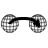Dynamic data structures are manipulated and traversed through pointers or object references. Programs that use dynamic data structures are thus expected to perform a greater number of object dereferences than a program which uses arrays as primary data structures; a basic metric can be developed from this observation. Of course a language such as Java which encourages object usage can easily skew this sort of measurement: an array-intensive program that stores array elements as objects (e.g., Complex objects) will result in as many object references as array references.
• data.pointerDensity.value: This metric gives a coarse indication of the importance of pointer references in a program. It is computed as the average number of loads of object references per kbc of executed code. The value 100 is used as a 7 threshold for considering a program likely to be pointer intensive. A continuous version, data.pointerDensity.continuous is also easily defined.

A potentially more accurate way of determining whether a program makes extensive use of dynamic, pointer-based data structures is to measure the graph diameter (length of longest path) of all data structures -- a very small diameter indicates very "flat" data structures, where pointer traversals will be shorter; a larger diameter indicates the need for deeper traversals to locate data. Again this approximate measure can be easily defeated by particular programming strategies: an array containing objects that maintain references to their immediate grid neighbours will have a relatively high diameter, whether or not the references are necessary or even used.

• data.pointerDiameter.value: This metric describes the length of the longest path of each weakly-connected data structure. Data structure roots can be approximated from the same root sets used by garbage collectors. Pointer polymorphism is typically measured as an average or maximum number of target addresses per pointer, and symmetrically number of pointers per target address (Cheng and Hwu argue that both are required for a more accurate measurement). This can be computed as a value metric; a bin version can also be appropriate, and is defined following.
• data.pointsToCount.value: This along with the symmetric data.pointsFromCount.value measures the average number of distinct objects referenced by each object references and the average number of object references directed at each object respectively. A continuous version can of course also be defined.
• data.pointsTo.bin: A pointer analysis system is most interested in identifying pointers that can be directed at one address, possibly two, but further divisions are often unnecessary. A bin metric can provide a more appropriate view in this case. Each bin gives the percentage of object references that referenced 1 object, 2 objects, and 3 or more objects. The symmetric companion bin, data.pointsFrom.bin, has bins for the percentage of objects that had 1, 2 or 3 or more references.